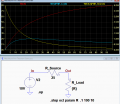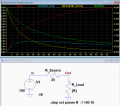# Maximum Power Transfer enlightenment needed.

#### Beetle_X

Joined Nov 2, 2012
67
The time has come where I need to understand this concept better but the guides I find on it seem to dance around the actual reasons.

I understand the viewpoint of the load as if the resistance is increased power can be increased because I and R are both part of what makes a load a load and are both factors mathematically. 5 x 4 is greater than 6 x 3 although one factor went down and the other when up.

The point that I can't see a connection on is the sources part in this all and how increasing impedance is going to get more power to the load or any connection on the impedance being equal.
Thanks for your expertise!Last edited:

#### crutschow

Joined Mar 14, 2008
32,932
If you solve the math for when the maximum power is given to the load for a fixed source resistance, you will find that it's when the load resistance equals the source resistance.

Note that this is different if you can change the source resistance.
In that case you would obviously set the source resistance to zero for the maximum load power.

Below is the LTspice simulation showing this:
The horizontal axis is the varying load resistance, the blue trace is its dissipated power versus resistance, the yellow trace is the source resistance power (both right vertical axis), and the red trace is the load voltage (left vertical axis).

You can see how the total power is shared between the load and source resistances, and that the peak load power occurs when the two resistances are equal, which causes the load voltage to be 1/2 the source voltage.

Make sense?Last edited:

#### Beetle_X

Joined Nov 2, 2012
67
I see the frame work for an example but no information. Let's say I have two resistance heaters in series in a simple circuit and one of the heaters will take the place of the source resistance. How does the one resistance effect the other in a positive way? It's just a matter of expending power at another point. The total power consumed is increased but the power used by the source or the other heater in this case does not act to increase the power of the other heater.
If this guide is used for reference , it clearly does not show how one effects the other and only shows that the source power consumed increases to only make the entire power consumption go up.

Last edited:

#### DickCappels

Joined Aug 21, 2008
10,068
In his first two sentences @crutschow gave you the key to your puzzlement.

If you solve the math for when the maximum power is given to the load for a fixed source resistance, you will find that it's when the load resistance equals the source resistance.

Note that this is different if you can change the source resistance.
In that case you would obviously set the source resistance to zero for the maximum load power.
Read it once or twice more and see if it makes sense, then look at his LTspice example again. It is all there.

#### crutschow

Joined Mar 14, 2008
32,932
I see the frame work for an example but no information.
There is a lot of information.
I'm sorry if you can't see it.
Is it Ohm's law you have a problem with?
How does the one resistance effect the other in a positive way?
What do you mean "in a positive way".
The maximum power theorem states that the maximum power you can generate in the load is when the load resistance is equal to the source resistance.
That's all is says, no more, no less.
It doesn't imply anything about one resistance affecting the other (other then in the relative power dissipated by each).

#### DickCappels

Joined Aug 21, 2008
10,068
The assumption is that you cannot adjust the resistance of the source, so what resistance of the load will cause the load to dissipate the most power.

Let's try an extreme example.

There is a 100 volt battery with a 100 ohm resistor in series is. The source resistance is fixed at 100 ohms. What value load resistor causes the greatest amount of power to be transferred to the load?

In the case of a zero ohm load, there is no voltage drop across the load, it is all across the source resistance, and therefore there is 0 watts dissipated in the load.

In the case of a 100 ohm load 50 volts is dropped across both the source resistance and the load resistance P=(V x V) R = 25 watts dissipated in the load.

In the case of a 120 ohm load, the current is 100V/220 ohms = 0.45 amps, and power in the load is I x I x R = 24.8 watts to the load. See either side of 100 ohms load resistance results in lower power being delivered to the load.

#### BobTPH

Joined Jun 5, 2013
7,561
Can you point me to the definition of the max power theorem you are using? I would like to know why you, and so many others keep getting wrong. Perhaps the wording of what you have read is at fault.

By the way, we get this question, in some form, often.

Bob

#### Beetle_X

Joined Nov 2, 2012
67
Thank you for all the replies!I did find a webpage that does seem to actually explain MPT and not just dance around the subject and repeat dogma. I'll get into what looks like to me as just excuses for a actual answer later in another post. Here's the link. https://hackaday.com/2015/07/29/say-it-with-me-input-impedance/ Scroll down to POWER TRANSFER.

In this guide and one other I found they disclose the missing factor which is to increase the voltage by dropping the current with impedance/resistance.Another way to look at this is the the voltage drop at the source is being reduced by reducing the current. It looks to do the the same thing as the combination of impedance and current does to the load separately which either brings power up or down with the right combination of the two. The only part I don't yet have an explanation for is why the impedance/resistance have be at a 1: 1 ratio.

Let me explain why this works with a easy example.
Let's say we have 1 dozen eggs in a egg container.There are 2 rows of 6 eggs which equals 12 of coarse. If there were 6 rows of 2 eggs it would represent the same amount because the mathematical factors in this case can be interchanged with the out come being the same.

let;s say either of the rows represent resistance or current. This is going to be a rough representation just to prove the point. If resistance is increased that means current has to go down and the combination of both current and resistance is going to equal 10 units in this example. 5 x 5 = 25. Both factors combined equal 10. 9 x 1.1 is close to 10 ( to make the explanation easy) but only represents 10 instead of 25 units. So in this way power can be increased although either resistance or current is being reduced .The same thing happens with increasing the entire resistance of a circuit but increasing resistance at the load.

Last edited:

#### crutschow

Joined Mar 14, 2008
32,932
why the impedance/resistance have be at a 1: 1 ratio.
Is that a philosophical question?
Look in Wikipedia for a math-based proof.

#### Beetle_X

Joined Nov 2, 2012
67
I did a edit and the edit posted the same time as your post. I apologize for that.

#### crutschow

Joined Mar 14, 2008
32,932
Didn't totally understand your egg analogy, but if it works for you, that's fine.

Perhaps this will also help a little.
Below is the simulation of the my circuit showing also the total power dissipated by both the source and load resistances (green trace) as delivered by the source.
Although the total power goes down as the load resistance increases, the maximum load power occurs when the two resistances (and thus powers) are equal.
Notice that the maximum power to the load is fairly flat with load resistance change, near the maximum point, so the exact value is not critical to achieving maximum load power.Last edited:

#### N5KS

Joined Apr 17, 2016
6
The time has come where I need to understand this concept better but the guides I find on it seem to dance around the actual reasons.

I understand the viewpoint of the load as if the resistance is increased power can be increased because I and R are both part of what makes a load a load and are both factors mathematically. 5 x 4 is greater than 6 x 3 although one factor went down and the other when up.

The point that I can't see a connection on is the sources part in this all and how increasing impedance is going to get more power to the load or any connection on the impedance being equal.
Thanks for your expertise!From reading your replies, it appears that you don’t understand the concept of ‘Source Resistance’. Think of a D cell battery for instance. It Is not only a source of voltage but it also has a source resistance that you CANNOT change, and your load will be in series with it. This concept of source voltage in series with source resistance applies to electronic circuits as well as batteries.